@Team 2018-02-03T06:50:17.000000Z 字数 13724 阅读 2084

# 目标检测算法之SSD

叶虎

## 前言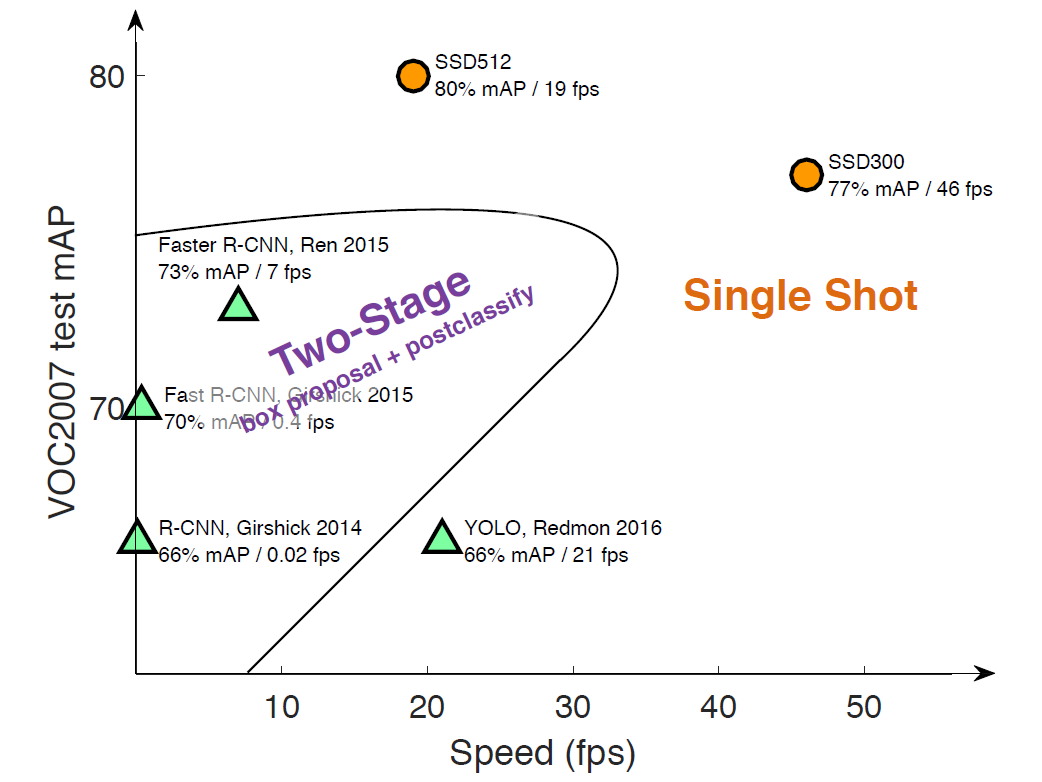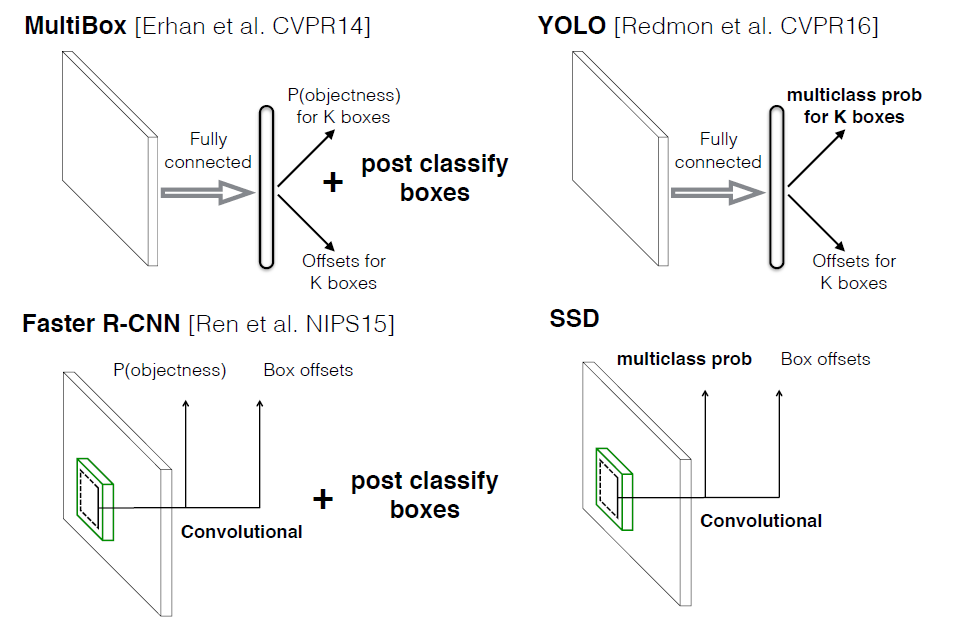## 设计理念

SSD和Yolo一样都是采用一个CNN网络来进行检测，但是却采用了多尺度的特征图，其基本架构如图3所示。下面将SSD核心设计理念总结为以下三点：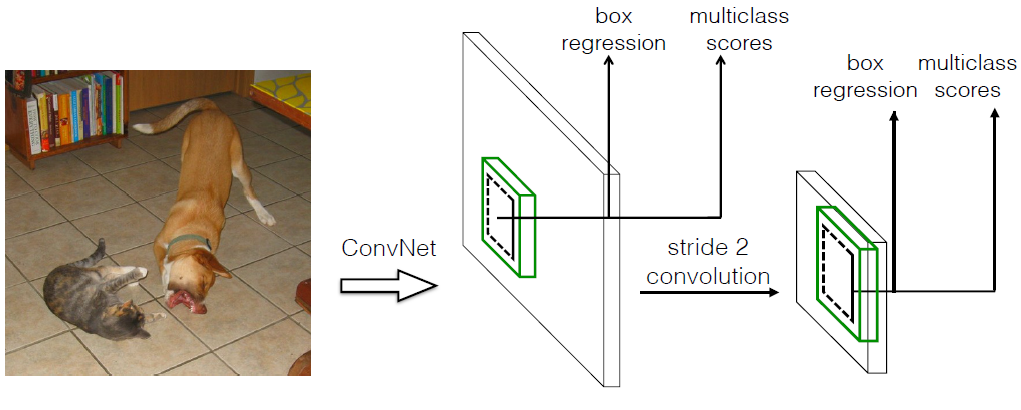（1）采用多尺度特征图用于检测（2）采用卷积进行检测

（3）设置先验框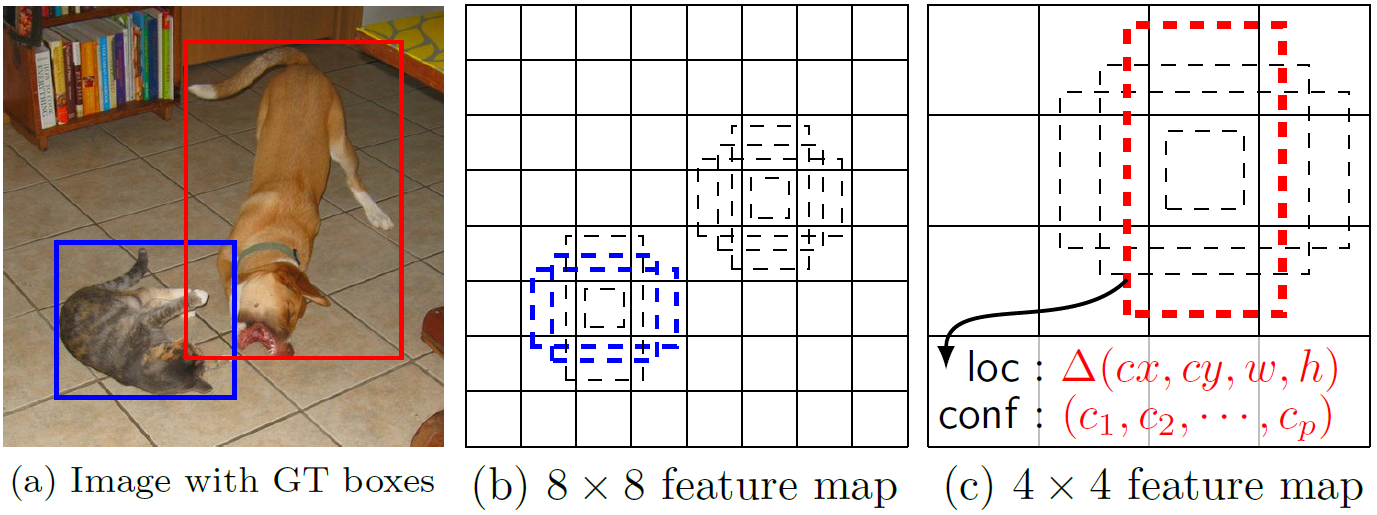SSD的检测值也与Yolo不太一样。对于每个单元的每个先验框，其都输出一套独立的检测值，对应一个边界框，主要分为两个部分。第一部分是各个类别的置信度或者评分，值得注意的是SSD将背景也当做了一个特殊的类别，如果检测目标共有$c$个类别，SSD其实需要预测$c+1$个置信度值，其中第一个置信度指的是不含目标或者属于背景的评分。后面当我们说$c$个类别置信度时，请记住里面包含背景那个特殊的类别，即真实的检测类别只有$c-1$个。在预测过程中，置信度最高的那个类别就是边界框所属的类别，特别地，当第一个置信度值最高时，表示边界框中并不包含目标。第二部分就是边界框的location，包含4个值$(cx, cy, w, h)$，分别表示边界框的中心坐标以及宽高。但是真实预测值其实只是边界框相对于先验框的转换值(paper里面说是offset，但是觉得transformation更合适，参见R-CNN）。先验框位置用$d=(d^{cx}, d^{cy}, d^w, d^h)$表示，其对应边界框用$b=(b^{cx}, b^{cy}, b^w, b^h)$表示，那么边界框的预测值$l$其实是$b$相对于$d$的转换值：

## 网络结构

SSD采用VGG16作为基础模型，然后在VGG16的基础上新增了卷积层来获得更多的特征图以用于检测。SSD的网络结构如图6所示。上面是SSD模型，下面是Yolo模型，可以明显看到SSD利用了多尺度的特征图做检测。模型的输入图片大小是$300\times300$（还可以是$512\times512$，其与前者网络结构没有差别，只是最后新增一个卷积层，本文不再讨论）。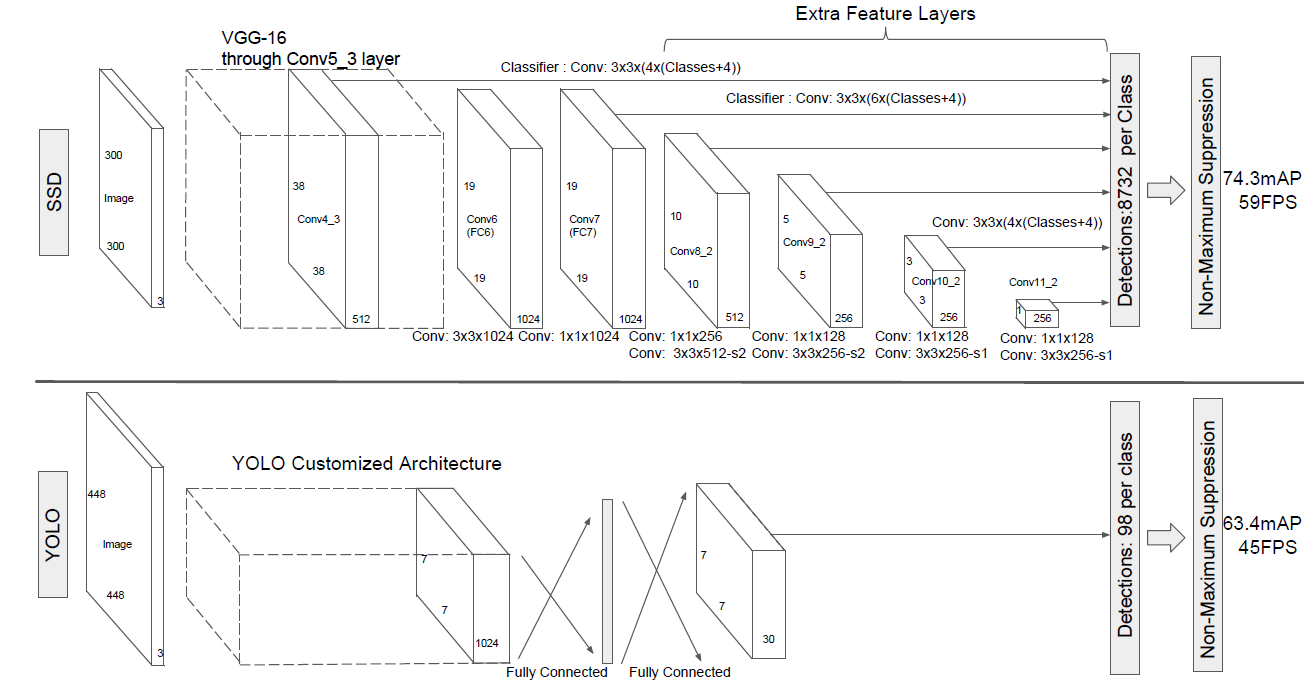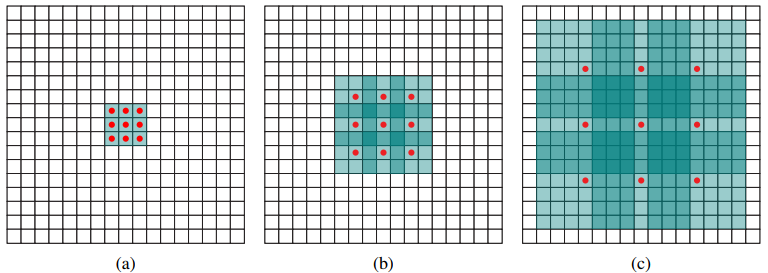# l2norm (not bacth norm, spatial normalization)
def l2norm(x, scale, trainable=True, scope="L2Normalization"):
n_channels = x.get_shape().as_list()[-1]
l2_norm = tf.nn.l2_normalize(x, , epsilon=1e-12)
with tf.variable_scope(scope):
gamma = tf.get_variable("gamma", shape=[n_channels, ], dtype=tf.float32,
initializer=tf.constant_initializer(scale),
trainable=trainable)
return l2_norm * gamma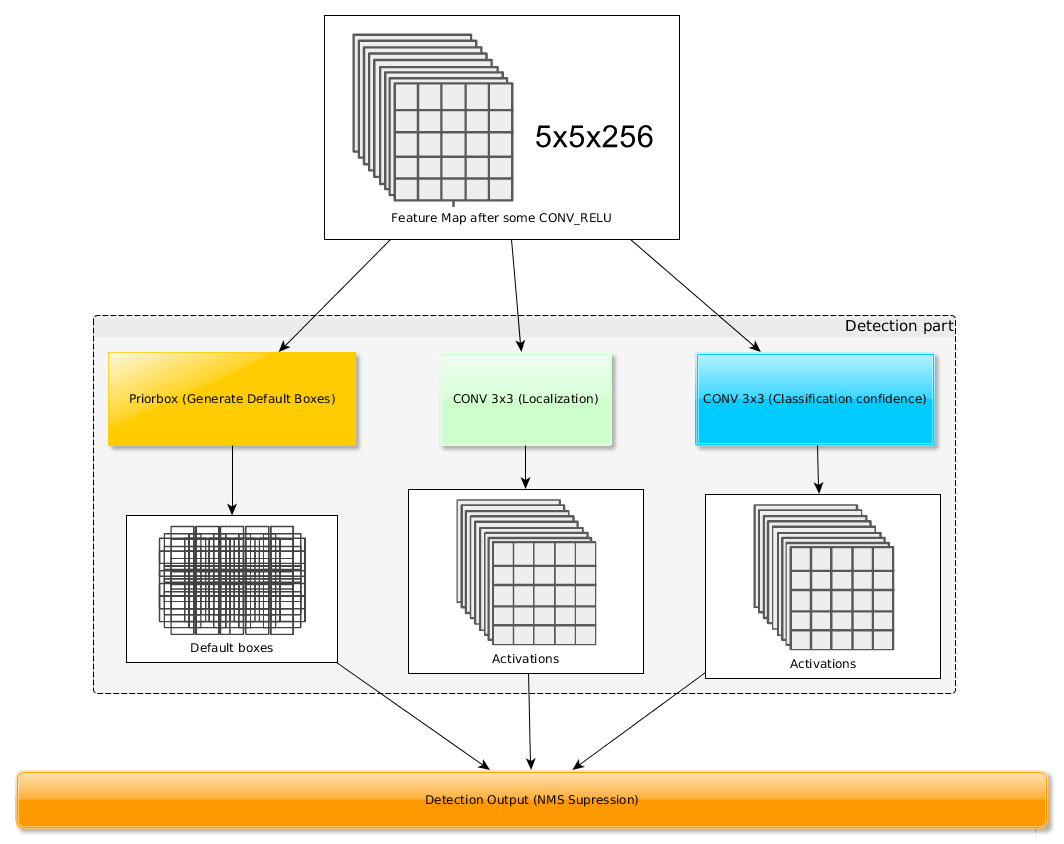## 训练过程

（1）先验框匹配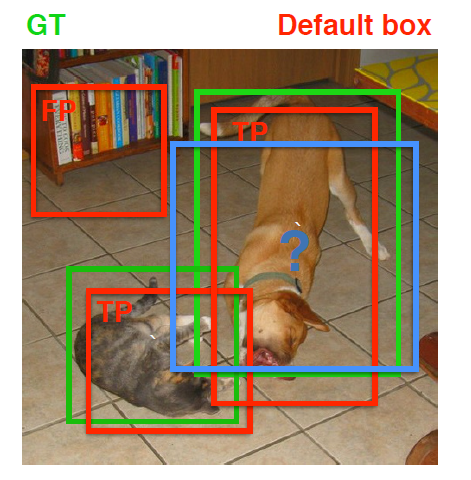（2）损失函数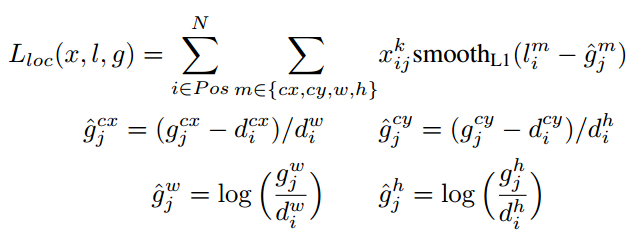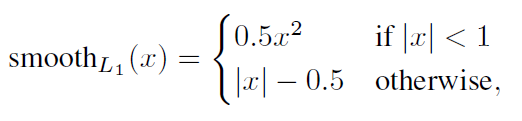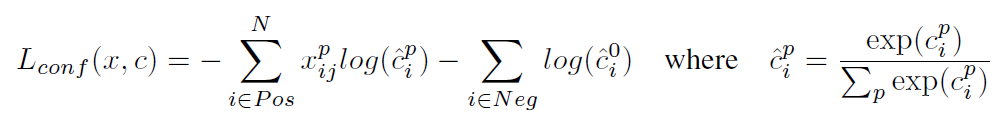（3）数据扩增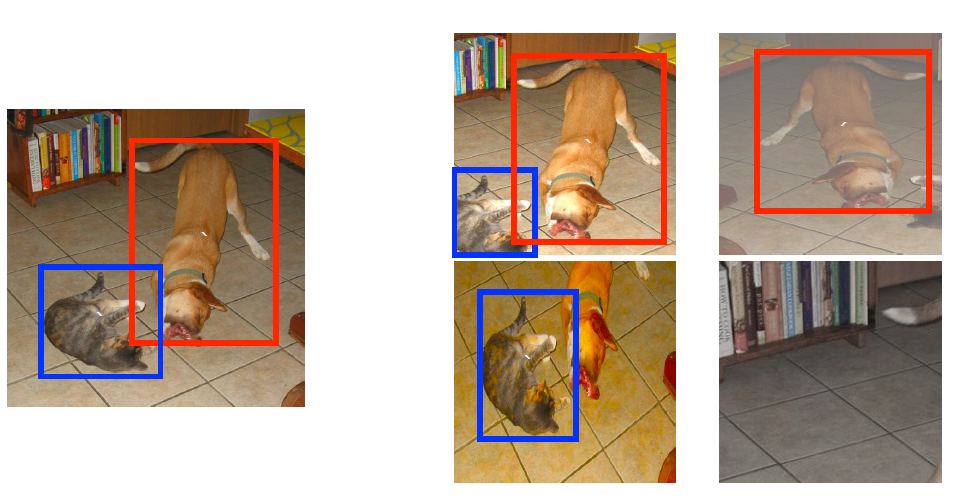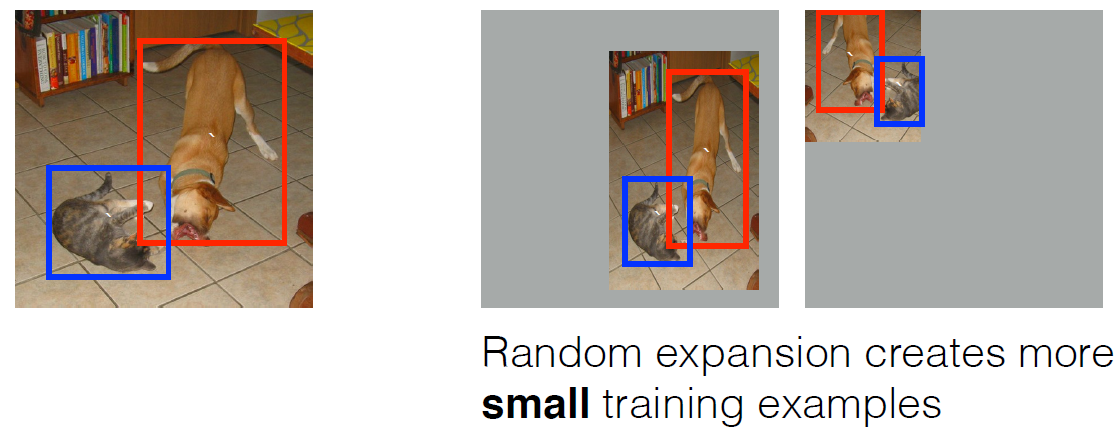## 性能评估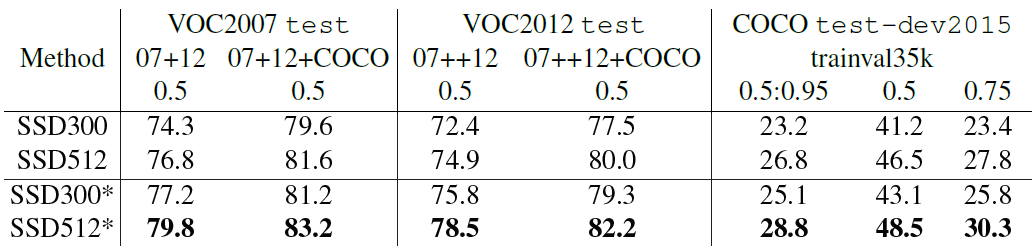SSD与其它检测算法的对比结果（在VOC2007数据集）如表2所示，基本可以看到，SSD与Faster R-CNN有同样的准确度，并且与Yolo具有同样较快地检测速度。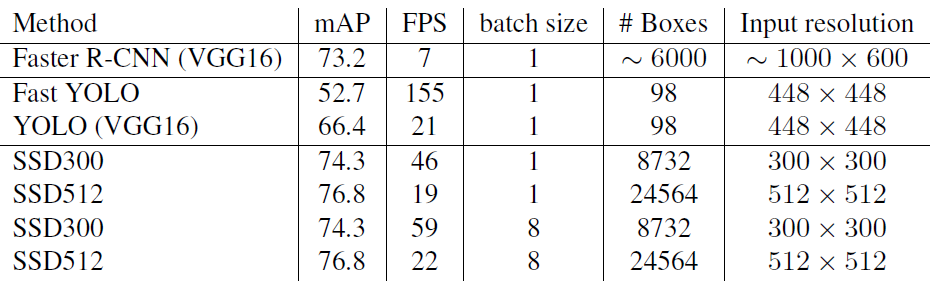• 数据扩增技术很重要，对于mAP的提升很大；
• 使用不同长宽比的先验框可以得到更好的结果；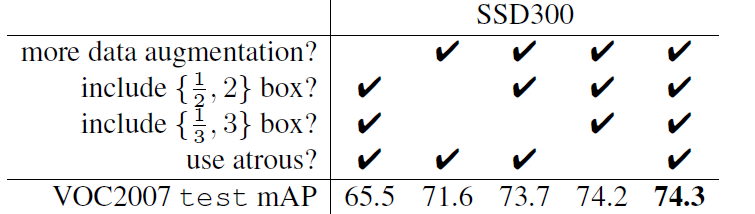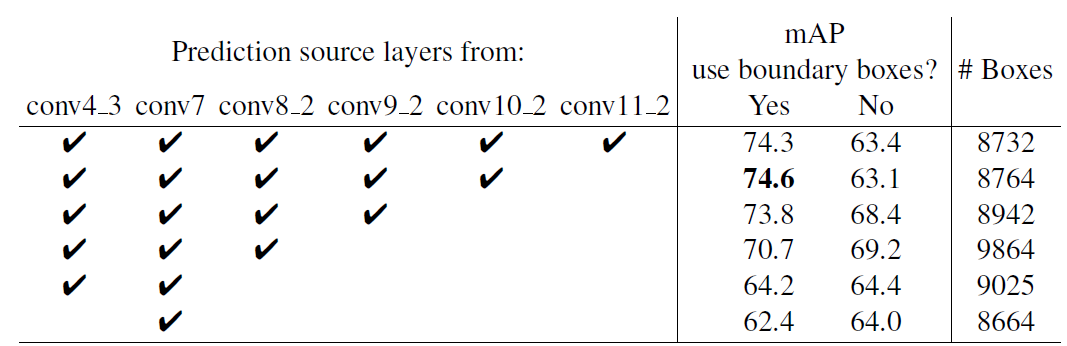## TensorFlow上的实现

SSD在很多框架上都有了开源的实现，这里基于balancap的TensorFlow版本来实现SSD的Inference过程。这里实现的是SSD300，与paper里面不同的是，这里采用$s_{min}=0.15, s_{max}=0.9$。首先定义SSD的参数：

self.ssd_params = SSDParams(img_shape=(300, 300),   # 输入图片大小
num_classes=21,     # 类别数+背景
no_annotation_label=21,
feat_layers=["block4", "block7", "block8", "block9", "block10", "block11"],   # 要进行检测的特征图name
feat_shapes=[(38, 38), (19, 19), (10, 10), (5, 5), (3, 3), (1, 1)],  # 特征图大小
anchor_size_bounds=[0.15, 0.90],  # 特征图尺度范围
anchor_sizes=[(21., 45.),
(45., 99.),
(99., 153.),
(153., 207.),
(207., 261.),
(261., 315.)],  # 不同特征图的先验框尺度（第一个值是s_k，第2个值是s_k+1）
anchor_ratios=[[2, .5],
[2, .5, 3, 1. / 3],
[2, .5, 3, 1. / 3],
[2, .5, 3, 1. / 3],
[2, .5],
[2, .5]], # 特征图先验框所采用的长宽比（每个特征图都有2个正方形先验框）
anchor_steps=[8, 16, 32, 64, 100, 300],  # 特征图的单元大小
anchor_offset=0.5,                       # 偏移值，确定先验框中心
normalizations=[20, -1, -1, -1, -1, -1],  # l2 norm
prior_scaling=[0.1, 0.1, 0.2, 0.2]       # variance
)


def _built_net(self):
"""Construct the SSD net"""
self.end_points = {}  # record the detection layers output
self._images = tf.placeholder(tf.float32, shape=[None, self.ssd_params.img_shape,
self.ssd_params.img_shape, 3])
with tf.variable_scope("ssd_300_vgg"):
# original vgg layers
# block 1
net = conv2d(self._images, 64, 3, scope="conv1_1")
net = conv2d(net, 64, 3, scope="conv1_2")
self.end_points["block1"] = net
net = max_pool2d(net, 2, scope="pool1")
# block 2
net = conv2d(net, 128, 3, scope="conv2_1")
net = conv2d(net, 128, 3, scope="conv2_2")
self.end_points["block2"] = net
net = max_pool2d(net, 2, scope="pool2")
# block 3
net = conv2d(net, 256, 3, scope="conv3_1")
net = conv2d(net, 256, 3, scope="conv3_2")
net = conv2d(net, 256, 3, scope="conv3_3")
self.end_points["block3"] = net
net = max_pool2d(net, 2, scope="pool3")
# block 4
net = conv2d(net, 512, 3, scope="conv4_1")
net = conv2d(net, 512, 3, scope="conv4_2")
net = conv2d(net, 512, 3, scope="conv4_3")
self.end_points["block4"] = net
net = max_pool2d(net, 2, scope="pool4")
# block 5
net = conv2d(net, 512, 3, scope="conv5_1")
net = conv2d(net, 512, 3, scope="conv5_2")
net = conv2d(net, 512, 3, scope="conv5_3")
self.end_points["block5"] = net
print(net)
net = max_pool2d(net, 3, stride=1, scope="pool5")
print(net)

# additional SSD layers
# block 6: use dilate conv
net = conv2d(net, 1024, 3, dilation_rate=6, scope="conv6")
self.end_points["block6"] = net
#net = dropout(net, is_training=self.is_training)
# block 7
net = conv2d(net, 1024, 1, scope="conv7")
self.end_points["block7"] = net
# block 8
net = conv2d(net, 256, 1, scope="conv8_1x1")
net = conv2d(pad2d(net, 1), 512, 3, stride=2, scope="conv8_3x3",
self.end_points["block8"] = net
# block 9
net = conv2d(net, 128, 1, scope="conv9_1x1")
net = conv2d(pad2d(net, 1), 256, 3, stride=2, scope="conv9_3x3",
self.end_points["block9"] = net
# block 10
net = conv2d(net, 128, 1, scope="conv10_1x1")
net = conv2d(net, 256, 3, scope="conv10_3x3", padding="valid")
self.end_points["block10"] = net
# block 11
net = conv2d(net, 128, 1, scope="conv11_1x1")
net = conv2d(net, 256, 3, scope="conv11_3x3", padding="valid")
self.end_points["block11"] = net

# class and location predictions
predictions = []
logits = []
locations = []
for i, layer in enumerate(self.ssd_params.feat_layers):
cls, loc = ssd_multibox_layer(self.end_points[layer], self.ssd_params.num_classes,
self.ssd_params.anchor_sizes[i],
self.ssd_params.anchor_ratios[i],
self.ssd_params.normalizations[i], scope=layer+"_box")
predictions.append(tf.nn.softmax(cls))
logits.append(cls)
locations.append(loc)
return predictions, logits, locations


# multibox layer: get class and location predicitions from detection layer
def ssd_multibox_layer(x, num_classes, sizes, ratios, normalization=-1, scope="multibox"):
pre_shape = x.get_shape().as_list()[1:-1]
pre_shape = [-1] + pre_shape
with tf.variable_scope(scope):
# l2 norm
if normalization > 0:
x = l2norm(x, normalization)
print(x)
# numbers of anchors
n_anchors = len(sizes) + len(ratios)
# location predictions
loc_pred = conv2d(x, n_anchors*4, 3, activation=None, scope="conv_loc")
loc_pred = tf.reshape(loc_pred, pre_shape + [n_anchors, 4])
# class prediction
cls_pred = conv2d(x, n_anchors*num_classes, 3, activation=None, scope="conv_cls")
cls_pred = tf.reshape(cls_pred, pre_shape + [n_anchors, num_classes])
return cls_pred, loc_pred


classes, scores, bboxes = self._bboxes_select(predictions, locations)


ssd_net = SSD()
classes, scores, bboxes = ssd_net.detections()
images = ssd_net.images()

sess = tf.Session()
# Restore SSD model.
ckpt_filename = './ssd_checkpoints/ssd_vgg_300_weights.ckpt'
sess.run(tf.global_variables_initializer())
saver = tf.train.Saver()
saver.restore(sess, ckpt_filename)

img = cv2.cvtColor(img, cv2.COLOR_BGR2RGB)
img_prepocessed = preprocess_image(img)   # 预处理图片，主要是归一化和resize
rclasses, rscores, rbboxes = sess.run([classes, scores, bboxes],
feed_dict={images: img_prepocessed})
rclasses, rscores, rbboxes = process_bboxes(rclasses, rscores, rbboxes)  # 处理预测框，包括clip,sort,nms

plt_bboxes(img, rclasses, rscores, rbboxes)  # 绘制检测结果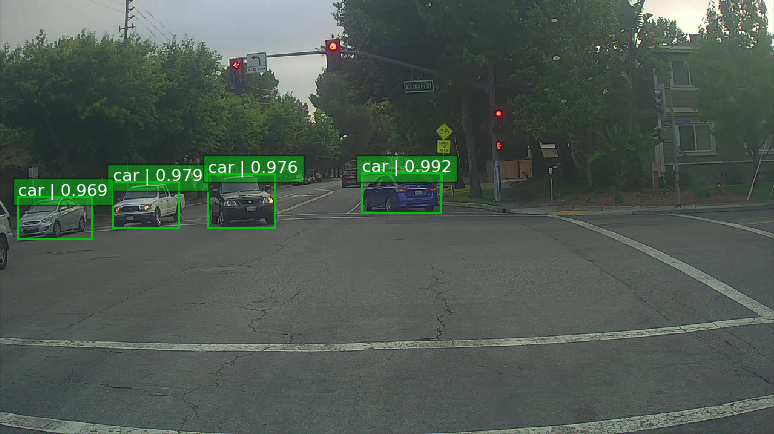## 小结

SSD在Yolo的基础上主要改进了三点：多尺度特征图，利用卷积进行检测，设置先验框。这使得SSD在准确度上比Yolo更好，而且对于小目标检测效果也相对好一点。由于很多实现细节都包含在源码里面，文中有描述不准或者错误的地方在所难免，欢迎交流指正。

## 参考文献• 私有
• 公开
• 删除